Log probability of model

Hello,

I am trying to reproduce Figure 2.29 from MBML chapter 2 showing the comparison of the log probabilities between two models and the log probabilities for each latent variable of interest.

What’s the best way to get the log probabilities from my model to make both panels shown in Figure 2.29?

I have seen other ways to get the log probability from this post, but I am seeing some errors.

1. potential_energy in MCMC.get_extra_fields results in an AttributeError: 'HMCGibbsState' object has no attribute 'potential_energy'
2. log_density Seems to work, but it only gives the total log probability, was expecting a dict from each site within the trace
3. log_density seems to work for model_00 but not my model_02 not sure why? Results in a ValueError: Incompatible shapes for broadcasting: ((4000, 22), (1, 48))

The code can be found in this notebook, but I will post both models below and the function call from 3, showing the error.

model_00
def model_00(
):
n_skills = max(map(max, skills_needed)) + 1

participants_plate = numpyro.plate("participants_plate", n_participants)

with participants_plate:
skills = []
for s in range(n_skills):
skills.append(numpyro.sample("skill_{}".format(s), dist.Bernoulli(0.5)))

for q in range(n_questions):
has_skills = reduce(operator.mul, [skills[i] for i in skills_needed[q]])
prob_correct = has_skills * (1 - prob_mistake) + (1 - has_skills) * prob_guess
isCorrect = numpyro.sample(
"isCorrect_{}".format(q),
dist.Bernoulli(prob_correct).to_event(1),
)
def model_02(
):
n_skills = max(map(max, skills_needed)) + 1

with numpyro.plate("questions_plate", n_questions):
prob_guess = numpyro.sample("prob_guess", dist.Beta(2.5, 7.5))

participants_plate = numpyro.plate("participants_plate", n_participants)

with participants_plate:
skills = []
for s in range(n_skills):
skills.append(numpyro.sample("skill_{}".format(s), dist.Bernoulli(0.5)))

for q in range(n_questions):
has_skills = reduce(operator.mul, [skills[i] for i in skills_needed[q]])
prob_correct = has_skills * (1 - prob_mistake) + (1 - has_skills) * prob_guess[q]
isCorrect = numpyro.sample(
"isCorrect_{}".format(q),
dist.Bernoulli(prob_correct).to_event(1),
)

nuts_kernel = NUTS(model_02)

kernel = DiscreteHMCGibbs(nuts_kernel, modified=True)

mcmc_02 = MCMC(kernel, num_warmup=200, num_samples=1000, num_chains=4)
mcmc_02.run(rng_key, jnp.array(responses), skills_needed)
mcmc_02.print_summary()

log_density_model_02 = log_density(model_02, (jnp.array(responses), skills_needed), dict(prob_mistake=0.1), mcmc_02.get_samples())

---------------------------------------------------------------------------
ValueError                                Traceback (most recent call last)
<ipython-input-16-ea553e8b7401> in <module>
----> 1 log_density_model_02 = log_density(model_02, (jnp.array(responses), skills_needed), dict(prob_mistake=0.1), mcmc_02.get_samples())

~/anaconda3/envs/numpyro_play/lib/python3.8/site-packages/numpyro/infer/util.py in log_density(model, model_args, model_kwargs, params)
51     """
52     model = substitute(model, data=params)
---> 53     model_trace = trace(model).get_trace(*model_args, **model_kwargs)
54     log_joint = jnp.zeros(())
55     for site in model_trace.values():

~/anaconda3/envs/numpyro_play/lib/python3.8/site-packages/numpyro/handlers.py in get_trace(self, *args, **kwargs)
163         :return: OrderedDict containing the execution trace.
164         """
--> 165         self(*args, **kwargs)
166         return self.trace
167

~/anaconda3/envs/numpyro_play/lib/python3.8/site-packages/numpyro/primitives.py in __call__(self, *args, **kwargs)
85             return self
86         with self:
---> 87             return self.fn(*args, **kwargs)
88
89

~/anaconda3/envs/numpyro_play/lib/python3.8/site-packages/numpyro/primitives.py in __call__(self, *args, **kwargs)
85             return self
86         with self:
---> 87             return self.fn(*args, **kwargs)
88
89

17     for q in range(n_questions):
18         has_skills = reduce(operator.mul, [skills[i] for i in skills_needed[q]])
---> 19         prob_correct = has_skills * (1 - prob_mistake) + (1 - has_skills) * prob_guess[q]
20         isCorrect = numpyro.sample(
21             "isCorrect_{}".format(q),

~/anaconda3/envs/numpyro_play/lib/python3.8/site-packages/jax/_src/numpy/lax_numpy.py in deferring_binary_op(self, other)
5867     if not isinstance(other, _scalar_types + _arraylike_types + (core.Tracer,)):
5868       return NotImplemented
-> 5869     return binary_op(self, other)
5870   return deferring_binary_op
5871

~/anaconda3/envs/numpyro_play/lib/python3.8/site-packages/jax/_src/numpy/lax_numpy.py in fn(x1, x2)
428 def _maybe_bool_binop(numpy_fn, lax_fn, bool_lax_fn, lax_doc=False):
429   def fn(x1, x2):
--> 430     x1, x2 = _promote_args(numpy_fn.__name__, x1, x2)
431     return lax_fn(x1, x2) if x1.dtype != bool_ else bool_lax_fn(x1, x2)
432   return _wraps(numpy_fn)(fn)

~/anaconda3/envs/numpyro_play/lib/python3.8/site-packages/jax/_src/numpy/lax_numpy.py in _promote_args(fun_name, *args)
324   _check_arraylike(fun_name, *args)
325   _check_no_float0s(fun_name, *args)
--> 326   return _promote_shapes(fun_name, *_promote_dtypes(*args))
327
328 def _promote_args_inexact(fun_name, *args):

~/anaconda3/envs/numpyro_play/lib/python3.8/site-packages/jax/_src/numpy/lax_numpy.py in _promote_shapes(fun_name, *args)
244       if config.jax_numpy_rank_promotion != "allow":
245         _rank_promotion_warning_or_error(fun_name, shapes)
247       return [broadcast_to(arg, (1,) * (result_rank - len(shp)) + shp)
248               for arg, shp in zip(args, shapes)]

~/anaconda3/envs/numpyro_play/lib/python3.8/site-packages/jax/_src/util.py in wrapper(*args, **kwargs)
184         return f(*args, **kwargs)
185       else:
--> 186         return cached(config._trace_context(), *args, **kwargs)
187
188     wrapper.cache_clear = cached.cache_clear

~/anaconda3/envs/numpyro_play/lib/python3.8/site-packages/jax/_src/util.py in cached(_, *args, **kwargs)
177     @functools.lru_cache(max_size)
178     def cached(_, *args, **kwargs):
--> 179       return f(*args, **kwargs)
180
181     @functools.wraps(f)

91   if result_shape is None:
---> 92     raise ValueError("Incompatible shapes for broadcasting: {}"
93                      .format(tuple(map(tuple, shapes))))
94   return result_shape

ValueError: Incompatible shapes for broadcasting: ((4000, 22), (1, 48))

From the docs, looking like you need "hmc_state.potential_energy". Could you double-check?

It doesn’t look like HMCGibbsState has a potential_energy attribute.

Can you show me an example to get the hmc_state? Maybe I am misunderstanding, but I am not running HMC, since the model has discrete latent sites, so I am not sure where to get an instance of hmc_state. If I do a Ctril+F for hmc_state on the doc page you linked I can only find one example obtaining a hmc_state using hmc with algo=NUTS.

Sorry, I meant to specify extra_fields=("hmc_state.potential_energy",). hmc_state is an attribute of HMCGibbsState, and potential_energy is an attribute of hmc_state. You can find other attributes of an hmc state here. Probably we should revise the docs to

current :data:~numpyro.infer.hmc.HMCState"

Do you want to add that enhancement?edit: never mind, I’ll make a PR to also fix some other docstrings.

So when I run with the extra fields shown below (using a single chain and few samples just to debug). I am missing the z key from HMCGibbsState and my hmc_state.z is also empty:

nuts_kernel = NUTS(model_00)

kernel = DiscreteHMCGibbs(nuts_kernel, modified=True)

mcmc = MCMC(kernel, num_warmup=10, num_samples=100, num_chains=1)
mcmc.run(rng_key, jnp.array(responses), skills_needed, extra_fields=("z", "hmc_state.potential_energy", "hmc_state.z"))
Output
mcmc.get_extra_fields()
{'hmc_state.potential_energy': DeviceArray([611.33057, 619.24493, 616.54944, 616.54944, 619.7796 ,
617.7407 , 620.2643 , 626.14923, 616.10535, 616.9027 ,
620.0015 , 619.3356 , 616.19617, 614.2479 , 619.55756,
616.59015, 619.2042 , 617.96277, 620.8396 , 619.07294,
619.1135 , 619.2042 , 618.40686, 623.6258 , 617.0341 ,
612.03723, 624.33246, 612.7439 , 622.253  , 615.75195,
620.13293, 615.7519 , 612.6126 , 616.7715 , 619.3356 ,
623.3631 , 615.17676, 614.47003, 617.0341 , 627.5627 ,
618.40674, 619.8202 , 622.43427, 618.05347, 615.17676,
612.2592 , 622.69696, 617.25616, 618.89154, 617.56885,
614.248  , 616.77136, 621.10236, 625.7957 , 620.2643 ,
613.5412 , 619.1135 , 614.6919 , 622.6063 , 619.20416,
621.80896, 613.9852 , 616.4587 , 616.4181 , 622.4751 ,
616.862  , 621.1023 , 617.1248 , 621.6777 , 617.8314 ,
619.1135 , 617.25616, 616.3274 , 614.2479 , 618.14417,
617.6094 , 621.6371 , 613.5414 , 622.697  , 612.96594,
621.415  , 621.85895, 621.7684 , 628.6227 , 616.4587 ,
614.692  , 618.89154, 612.6125 , 628.6227 , 633.13495,
622.4343 , 620.04224, 619.6889 , 613.89453, 621.9905 ,
627.4719 , 618.2756 , 619.91095, 625.4425 , 616.9027 ],            dtype=float32),
'hmc_state.z': {}}

Ah, it took me a minute to see how to use the interface. Maybe this could be in the docs as well, showing an explicit:

mcmc.run(..., extra_fields=("hmc_state.potential_energy",))

On my first few reads, I didn’t understand how to get the extra information. With your help and iterating through the errors, I started to realize the extra fields need to align with the attributes/fields of the named tuples shown in the docs.

Thank you for the offer and I am glad you got it. =)

I had a little more time to look over the docs and source code. I believe this is expected behavior since, latent variable fields are returned by default (?). So these samples are just within mcmc.get_samples(). Still, I am unsure how/what/why z in extra_fields could be used.

Is -potential_energy from mcmc.run(..., extra_fields="hmc_state.potential_energy") the log density of the joint posterior?

Is there a way to get a marginal log density of the posterior for specified latent sites?

Still thinking about how to reproduce the figure 2.29 (b) shown below. I am guessing Figure 2.29 (b) is a marginal posterior log density (?) since the parts don’t sum to the whole shown in Figure 2.29(a).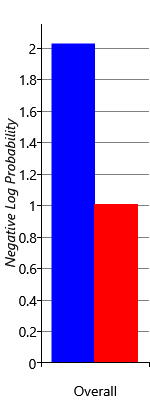Figure 2.29(a)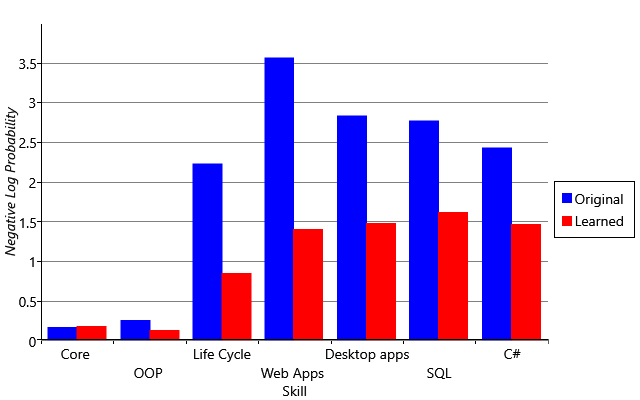Figure 2.29(b)

That’s right up to unconstrained transforms (of continuous latent variables with constrained supports like positive).

marginal log density of the posterior for specified latent sites

Could you clarify what you are looking for? Some formula would be helpful. From the figures, I can see that the y column is negative of log probability. Not sure what is your latent variables though.

If you want to get the marginalized posterior distribution over some discrete latent variables, then you just draw a large number of samples and plot the (normalized if necessary) histogram. Then you can convert the histogram result (i.e. prob(x=i) for each i) to some sort of “negative log probability” if you want: negative_log_prob(x=i) = -log(prob(x=i)).

Sorry for not clearifying.

In Figure 2.29(b) each bar is the latent variable of interest where the skill (Core, OOP, Life Cycle, etc.) ~Berr(\theta_i). The color indicates two model iterations, blue was model_00 and red was model_02 from my OP, reproduced below for convenience.

model_00
def model_00(
):
n_skills = max(map(max, skills_needed)) + 1

participants_plate = numpyro.plate("participants_plate", n_participants)

with participants_plate:
skills = []
for s in range(n_skills):
skills.append(numpyro.sample("skill_{}".format(s), dist.Bernoulli(0.5)))

for q in range(n_questions):
has_skills = reduce(operator.mul, [skills[i] for i in skills_needed[q]])
prob_correct = has_skills * (1 - prob_mistake) + (1 - has_skills) * prob_guess
isCorrect = numpyro.sample(
"isCorrect_{}".format(q),
dist.Bernoulli(prob_correct).to_event(1),
)
model_02
def model_02(
):
n_skills = max(map(max, skills_needed)) + 1

with numpyro.plate("questions_plate", n_questions):
prob_guess = numpyro.sample("prob_guess", dist.Beta(2.5, 7.5))

participants_plate = numpyro.plate("participants_plate", n_participants)

with participants_plate:
skills = []
for s in range(n_skills):
skills.append(numpyro.sample("skill_{}".format(s), dist.Bernoulli(0.5)))

for q in range(n_questions):
has_skills = reduce(operator.mul, [skills[i] for i in skills_needed[q]])
prob_correct = has_skills * (1 - prob_mistake) + (1 - has_skills) * prob_guess[q]
isCorrect = numpyro.sample(
"isCorrect_{}".format(q),
dist.Bernoulli(prob_correct).to_event(1),
)

I am looking for opinions on how/what is Figure 2.29(b) is plotting? Because I don’t understand how to obtain a similar figure from the fitted model. I was hoping someone with more experience would recognize how to make the figure. Figure 2.29(a) makes sense to me since it seems to be the log density of the model.

My term of marginal log density of the posterior for specified latent sites is my guess as what Figure 2.29(b) is plotting. 🤷

I will give that a try.

If x axis is values of your discrete latent variables, then it makes sense to use histogram as in my last comment. Please let me know if it works.You were spot on, and I was able to reproduce Figure 2.29. The key detail was the figure displays the negative log probability of the ground truth, where the ground truth is an indicator variable for each skill for each participant. Each participant was asked which development skills they consider that they have. So the figure was just the mean of:

−log(p(skill_i=truth_i)) with p(skill_i) ~ Bernoulli(θ_i)

Which is calculated with a little helper function, shown below. I had to add machine \epsilon using np.finfo(float).eps to avoid the inf log(p) when p = 0. But the figures look good at first glance.

neg_log_proba_score
def neg_log_proba_score(posterior_samples: Dict, params_sites: List[str], y_true):
"""
Calculates the the negative log probability of the ground truth, the self assessed skills.
:param posterior_samples Dict: dictionary of samples from the posterior.
:param params_sites List[str]: a list of params to compute proba
:y_true array-like dtype == int: array of indicator variables for skill of participants
"""
assert np.issubdtype(y_true.dtype, np.integer)
proba = np.zeros((len(params_sites), posterior_samples[params_sites].shape[-1]))
assert proba.shape == y_true.shape
for i, param in enumerate(params_sites):
proba[i, :] = np.mean(posterior_samples[param], axis=0)

score = scipy.stats.bernoulli(proba).pmf(y_true)
score[score == 0.0] = np.finfo(float).eps

return -np.log(score)

I have updated the notebook, and you can see the update at the nbviewer link here

Edit: I reproduce a similar trend of the learned model (model_02) score is lower than the original (model_00), but I am noticing larger differences between my negative log probability of the ground truth scores and the MBML’s result. If there aren’t any bugs, I wonder if you can attribute the differences to just the inference used?

MBMLMine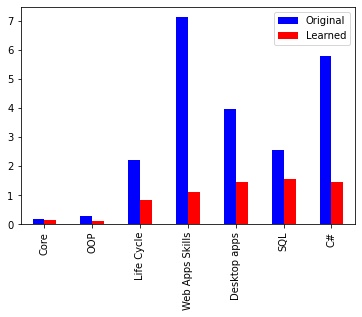It would be nice to use plates as in the tutorial. It is sad if we can’t translate 1-1 from a graphical model to a numpyro model.I guess you can do something like

skills_needed = ...  # boolean with shape num_questions x num_skills
with questions_plate:
prob_guess = ...

with plate("people", ..., dim=-2):
with skills_plate:
skill = ...

with questions_plate:
# shape: people x questions x skills
relevant_skills = skill[:, None, :] | (~skills_needed)
# shape: people x questions
has_skill = jnp.all(relevant_skills, -1)
prob_correct = ...
is_correct = ...

After verifying that the plate diagrams are consistent, I guess it is easier to spot the issue.

Sorry for the long pause.

Thank you for the code of the question_plate. I wasn’t really sure how to vectorize before your help.

Here are the model diagrams with plates, model_03 is without learning the guessing probability and model_04 is with learning the guessing probability. It looks like I reproduce the results from the book with model_04 but model_03 still looks off from the original since the negative log probability of the ground truth still is larger than the book. I don’t see anything obviously wrong, but I am happy to have the vectorized version. =)

model_03
def model_03(
):
n_skills = skills_needed.shape

questions_plate = numpyro.plate("questions_plate", n_questions)

# skills.shape == (n_participants, n_skills)
with numpyro.plate("participants_plate", n_participants, dim=-2):
with numpyro.plate("skills_plate", n_skills):
skills = numpyro.sample("skill", dist.Bernoulli(0.5))

with questions_plate:
# shape: people x questions x skills
# astype(bool) is needed for the log density
relevant_skills = skills[:, None, :].astype(bool) | (~skills_needed)
# shape: people x questions
has_skill = jnp.all(relevant_skills, -1)
prob_correct = has_skill * (1 - prob_mistake) + (1 - has_skill) * prob_guess
is_correct = numpyro.sample(
)
model_04
def model_04(
):
n_skills = skills_needed.shape

questions_plate = numpyro.plate("questions_plate", n_questions)

with questions_plate:
prob_guess = numpyro.sample("prob_guess", dist.Beta(2.5, 7.5))

# skills.shape == (n_participants, n_skills)
with numpyro.plate("participants_plate", n_participants, dim=-2):
with numpyro.plate("skills_plate", n_skills):
skills = numpyro.sample("skill", dist.Bernoulli(0.5))

with questions_plate:
# shape: people x questions x skills
# astype(bool) is needed for the log density
relevant_skills = skills[:, None, :].astype(bool) | (~skills_needed)
# shape: people x questions
has_skill = jnp.all(relevant_skills, -1)
prob_correct = has_skill * (1 - prob_mistake) + (1 - has_skill) * prob_guess
is_correct = numpyro.sample(
)

model_03: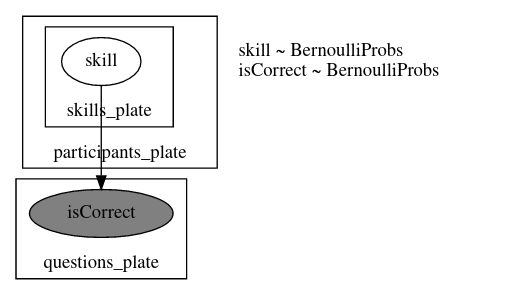model_04:

Figure 2.29(a) with plates: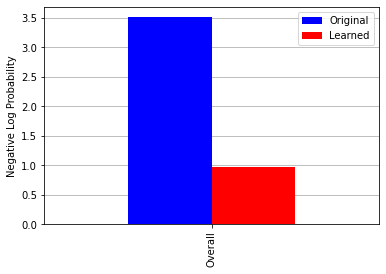Figure 2.29(b) with plates: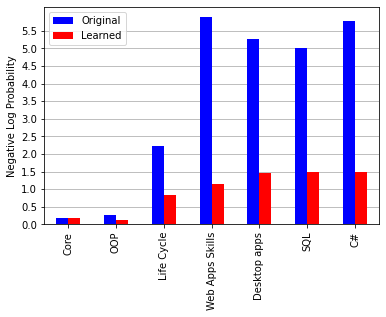Edit: added Figure 2.29(a) with plates

Probably not important but it is better to let isCorrect be in the participants_plate. You might also want to use -dist.Bernoulli(p).log_prob(y) instead of neg_log_proba_score(p, y) (the numerical bug seems to be fixed in the master branch https://github.com/pyro-ppl/numpyro/pull/1108). Other than that, both modeling and inference codes look reasonable to me. You might also want to double-check if all the procedure is the same as in the book (e.g. model input, drawing code,…)

Edit: How about removing modified=True? I think using modified Gibbs is not good for Bernoulli in general, especially for Bernoulli(0.5) (under modified Gibbs, 0 → 1 and 1 → 0 for every Gibbs step, which is periodic).